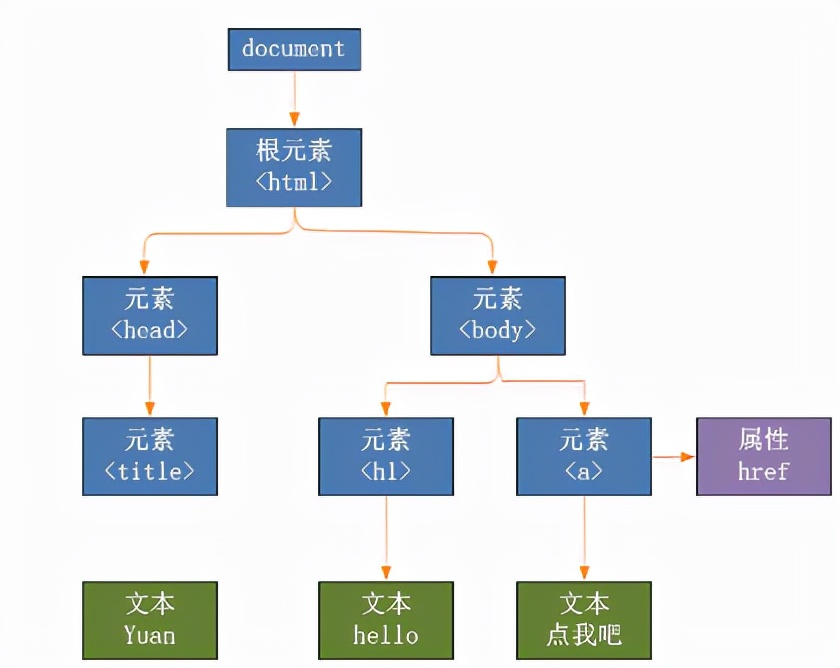##全国校区# 提取任意网页核心内容——像搜索引擎一样精准2021-10-26

# 提取文章内容

```divs = response.xpath("body//div")
sel = None
maxvalue = 0 for d in divs:
ds = len(d.xpath(".//div"))
ps = len(d.xpath(".//p")) value = ps - ds if value > maxvalue:
sel = { "node": d, "value": value }
maxvalue = value print("".join(sel['node'].getall())) ```
• response 是页面的一个响应，其中包含了页面的所有内容，可以通过 xpath 提取想要的部分
• "body//div" 的意思是提取所以 body 标签下的 div 子标签，注意：// 操作是递归的
• 遍历所有提取到的标签，计算其中包含的 div 数量，和 p 数量
• p 数量 和 div 数量的差值作为这个元素的权值，意思是如果这个元素里包含了大量的 p 时，就认为这里是文章主体
• 通过比较权值，选择出权值最大的元素，这便是文章主体
• 得到文章主体之后，提取这个元素的内容，相当于 jQuery 的 outerHtml

1. 有的文章内容被放在了 <article> 标签里了，所以没有获取到
2. 有的文章每个 <p> 外面都包裹了一个 <div>，所以 p 的数量 和 div 的抵消了

```divs = response.xpath("body//*")
sels = []
maxvalue = 0 for d in divs:
ds = len(d.xpath(".//div"))
ps = len(d.xpath(".//p")) if ps >= maxvalue:
sel = { "node": d, "ps": ps, "ds": ds
}
maxvalue = ps
sels.append(sel)

sels.sort(lambda x: x.ds)

sel = sels print("".join(sel['node'].getall()))```
• 方法主体里，先挑选出 p 数量比较大的节点，注意 if 判断条件中 换成了 >= 号，作用时筛选出同样具有 p 数量的结点
• 经过筛选之后，按照 div 数量排序，然后选取 div 数量最少的

# 选择最优```def find(node, sel): value = 0 for n in node.xpath("*"): if n.xpath("local-name()").get() == "p":
t = "".join([s.strip() for s in (n.xpath('text()').getall() + n.xpath("*/text()").getall())]) value += len(t) else: value += find(n, a)*0.5 if value > sel["value"]:
sel["node"] = node
sel["value"] = value return value sel = { 'value': 0, 'node': None
}
find(response.xpath("body"), sel)```
• 定义了一个 find 函数，这是为了方便做递归，第一次调用的参数是 body 标签，和前面一样
• 进入方法里，只找出该节点的直接孩子们，然后遍历这些孩子
• 判断如果孩子是 p 节点，提取出其中的所有文字，包括子节点的，然后将文字的长度作为权值
• 提取文字的地方比较绕，先取出直接的文本，和间接文本，合成 list，对每部分文本做了去除前后空字符，最后合并为一个字符串，得到了所包含的文本
• 如果孩子节点不是 p，就递归调用 find 方法，而 find 方法返回的是 指定节点所包含的文本长度
• 在获取子节点的长度时，做了缩减处理，用以体现距离越远，权值越低的规则
• 最终通过 引用传递的 sel 参数，记录权值最高的节点

50% 的坡度比率是如何得到的呢？

OK